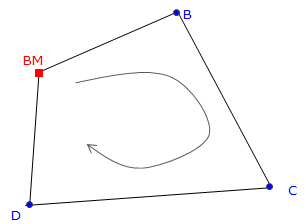## 1. Principles

Trigonometric leveling is so named because it uses a total station instrument's (TSI) slope distance and zenith angle measurements to mathematically compute an elevation difference which, with a few more bits of information, can be used to determine a point's elevation. Using appropriate procedures, and controlling errors, elevation accuraciy can be better than 0.1 ft. Because trigonometric leveling is not limited to a horizontal line of sight, it is more flexible and provides faster elevation data collection than differential leveling.

### a. Elevation determination

TSI slope reduction is discussed in the Electronic Distance Measurement topic. Slope reduction geometry is shown in Figure F-1 and accompanying Equations F-1 and F-2.Figure F-1 Trigonometric RelationshipsEquation F-1Equation F-2 H: Horizontal distance Z: Zenith angle S: slope distance V: Vertical distance R: Earth radius k: Refraction constant

The terms in brackets in both equations account for earth curvature and atmospheric refraction. These are systematic errors which are compensated mathematically. The refraction constant is generally taken as a percentage of earth curvature. At low to medium altitudes k = 0.14 (14%), at higher altitudes k=0.07 due to a thinner atmosphere. Earth radius, R, is 20.906x106 ft

For short distances, earth curvature and refraction drop out and the equations become simplified. Most TSI have the option to turn on the corrections.

Vertical distance, V, is the elevation difference between the TSI and the reflector. Adding V to the TSI elevation gives us the reflector elevation. What we want, however is the elevation of the ground point at the reflector location. To determine that, we need two additional pieces of information: (1) TSI elevation, and, (2) height of the reflector above the ground point. Once we have those, then the elevation of any observed point, i, is computed from:

 Elevi = ElevTSI + Vi - HRi Equation F-3 ElevTSI: Elevation of the TSI HRi: Height of reflector at point i

### b. Sideshots

Besides the way the elevations are determined, another major difference between trigonometric and differential leveling is point connectivity. In differential leveling we normally have one BS and one FS at each set up - we survey in then out of each elevation point. Trigonometric leveling is used when a number of elevations are measured from a single instrument set up. All those points are surveyed into, but not out of. Each elevation point determined by trigonometric leveling is an open link, also known as a sideshot.

In a closed differential level network, Figure F-2(a), each point has a BS and FS; each is connected to another point and their elevations are based on the BM.

Figure F-2(b) depicts a trigonometric network referenced to the differential network. Points B, C, and D serve as control for trigonometric leveling. The green shots at points B, C, and D are all sideshots. Because sideshots are not connected to other points their elevations cannot be checked. An elevation error could be in an individual sideshot measurement or a control point elevation error which affects all the sideshots from that point(a) Control Network (b) Sideshots Figure F-2 Control Network and Sideshots